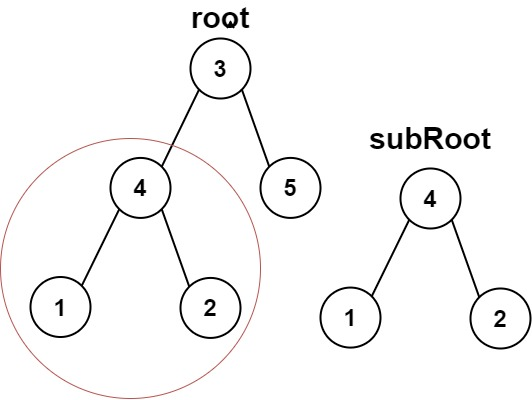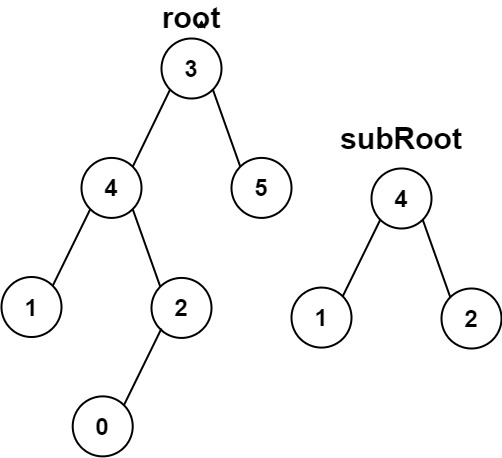### LeetCode• ㊗️
• 大家
• offer
• 多多！

## Problem

Given the roots of two binary trees root and subRoot, return true if there is a subtree of root with the same structure and node values of subRoot and false otherwise.

A subtree of a binary tree is a tree that consists of a node in tree and all of this node’s descendants. The tree could also be considered as a subtree of itself.

Example 1:Input: root = [3,4,5,1,2], subRoot = [4,1,2]
Output: true


Example 2:Input: root = [3,4,5,1,2,null,null,0], subRoot = [4,1,2]
Output: false


Constraints:

• The number of nodes in the root tree is in the range [1, 2000].
• The number of nodes in the subRoot tree is in the range [1, 1000].
• $-10^4 <= root.val <= 10^4$
• $-10^4 <= subRoot.val <= 10^4$

## Code

class Solution {
public boolean isSubtree(TreeNode root, TreeNode subRoot) {
if (subRoot == null) return true;
if (root == null) return false;
if (root.val == subRoot.val) {
if (helper(root, subRoot)) {
return true;
}
}

return isSubtree(root.left, subRoot) || isSubtree(root.right, subRoot);
}

private boolean helper(TreeNode root, TreeNode subRoot) {
if (root == null && subRoot == null) return true;
if (root == null || subRoot == null) return false;

if (root.val != subRoot.val) return false;

return helper(root.left, subRoot.left) && helper(root.right, subRoot.right);
}
}